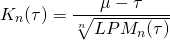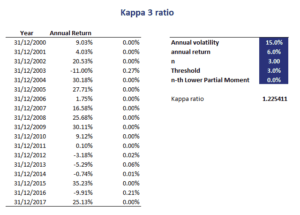# Kappa ratio

The Kappa ratio (KR), more commonly referred to as the Kappa 3 ratio, is a measure of risk-adjusted performance that considers downside risk. The Kappa measure was introduced by Kaplan and Knowles in 2004. The ratio is mainly used to rank investments in terms of attractiveness. The advantage of the Kappa measure, which the Kappa 3 ratio shares with the Omega ratio and Sortino ratio, is that the ratios do not assume returns are normally distributed. This is different from the Sharpe ratio, which assume returns are normally distributed.

Here, we provide the Kappa ratio definition, discuss the interpretation of the Kappa 3 ratio, and finally provide an example in Excel that implements the calculation. We also discuss how both the Omega ratio and Sortino ratio can be derived from the Kappa.

## Kappa ratio definition

Let’s have a look at the Kappa ratio formula:Where mu is the mean return, tau is the threshold return, and LPMn is the n-th order Lower Partial Moment.

When we set the parameter j to 1, we get the Omega ratio – 1. So, adding 1 we get the Omega ratio. When we set j to 2, we get the Sortino ratio. In other words, the ratio unifies the Sortino and Omega ratio. The most commonly used value for j is 3. This explain why the ratio is generally referred to as the Kappa 3 ratio.

## Kappa ratio interpretation

The higher the kappa measure, the better. Unfortunately, differences in Kappa values are hard to interpret. Thus, when using the kappa measure, be should use it as an ordinal scale. Thus, we only use the Kappa ratio to rank investments

## Kappa ratio example

Next, let’s consider a simple example to illustrate the calculation of the Kappa ratio in Excel. The following figure shows what the calculations look like. To calculate the ratio, we first need to be able to calculate the Lower Partial Moments. We discuss the calculation of Lower Partial Moments on this page.The Kappa 3 ratio spreadsheet calculator itself, which implements the kappa ratio example, can be downloaded at the bottom of the page.

## Summary

We discussed the Kappa measure, a measure of risk-adjusted performance which relies on downside risk. In particular, the kappa makes use of Lower Partial Moments as the measure or risk, instead of the more commonly used measure of risk standard deviation.

### Kappa calculation

Want to have an implementation in Excel? Download the Excel file: Kappa Ratio template